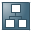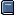Adjustment of Interest-Bearing AccountsPurpose

This process describes how you can adjust for inflation interest-bearing accounts (in the SAP System, G/L accounts with interest indicators) so that any interest earned is reduced to reflect the general inflation index.

Prerequisites

In addition to the usual settings required for adjusting a G/L account, you must also bear in mind the following:

• Since the system only supports the use of the general inflation index, you must not have assigned any interest-bearing accounts a specific index.
• In the account determination settings for the account to be adjusted, you must have entered the interest earned account as the general inflation contra account. This enables the system to deduct the inflation from the interest earned.

Process Flow

1. You calculate the interest accrued on each G/L account.
2. To do so, you run theinterest calculation program as normal. The program calculates the interest accrued and posts it to the interest receivable and interest earned accounts that you have defined in the program's Customizing settings.

3. You calculate the inflation on each G/L account.

You do so using the Inflation Adjustment of G/L Accounts program. This calculates the inflation on all accounts, using the general inflation index, and transfers the inflation from the interest earned account to the general inflation gain or loss account.

Example

You have a bank account with a balance of UNI 100,000. The interest rate is 10%, and the general inflation rate is running at 3%.

1. You run the interest calculation program, and the system calculates the interest (UNI 10,000), which it posts to the interest receivable and interest earned accounts (in the graphic below, posting 1).
2. You run the Inflation Adjustment of G/L Accounts program, and the system calculates the inflation on the bank account over the period (UNI 3,000) and posts it to the interest earned account and the inflation gain or loss account (2).

This effectively reduces the interest earned from UNI 10,000 to UNI 7,000.